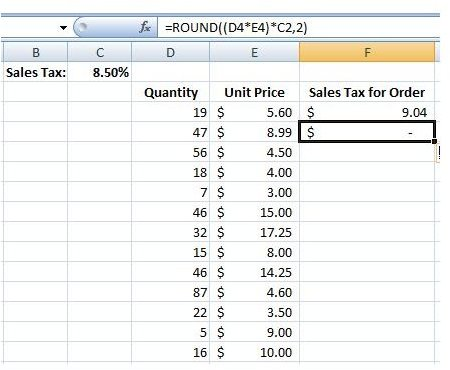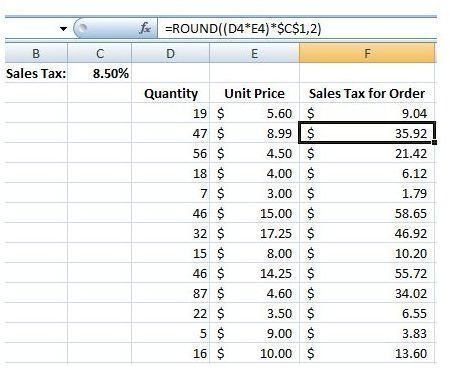# How to Copy a Formula with a Fixed Cell Reference in Excel

Page content

## Copying an Excel Formula with a Fixed Value

Problem: You have 5,000 rows of data. As shown in Fig. 173 (click any image for a larger view), each row contains a quantity and the unit price. The sales tax rate for all orders is shown in cell C1. After entering a formula to calculate the total sales tax for the order in the first row, how do you copy the formula down to other rows?

## The Solution

If you copy the formula in F4 to F5, you get an invalid result, as shown in Fig. 174 below. This is a standard result that occurs when copying formulas with relative references.Look at the formula in the formula bar in Fig. 174. As you copied the formula, the references to D4 and E4 changed as expected. However, the reference to C1 moved to C2. You need to find a way to copy this formula and always have the formula reference C1.

Frankly, this is the most important technique in the entire book. I once had a manager who would enter every formula by hand in the entire dataset. I didn’t have the heart to tell him there was an easier way.

Strategy: You need to indicate to Excel that the reference to C1 in the formula is Absolute. Do this by inserting a dollar sign before the C and before the 1 in the formula. The formula in F4 would change to =ROUND((D4*E4)*\$C\$1,2). As you copy this formula down to other rows in your dataset, the portion that refers to \$C\$1 will continue to point at \$C\$1, as shown in Fig. 175 below.Additional Details: In another tutorial, we’ll cover material that will help to understand the effect of using just one dollar sign in a reference instead of two. Read Simplify Entry of Dollar Signs in Formulas a few chapters after that to learn a cool shortcut for entering the dollar signs automatically.

Summary: Entering dollar signs in a reference will lock the reference and make it absolute, or fixed. No matter where you copy the formula, it will continue to point to the original cell with the fixed value.

Functions Discussed: =ROUND()

Related Resources:

• Create a Multiplication Table in Excel – This tutorial gives an example of when you would only want to use one dollar sign in a cell reference, keeping only the row or the column fixed.

• 91 Tips for Calculating with Microsoft Excel – Looking for Excel help and not sure what words to search for? This directory links to 91 individual tips and tricks for overcoming common Excel problems. Written by Bill Jelen (otherwise known as Mr. Excel), this collection of short, easy tutorials will help you improve your Excel skills in no time.

• Basic Microsoft Excel Cheat Sheet – Learn quick keyboard shortcuts and find out more about how to use some of the most helpful Excel functions.